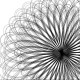Don't Miss

# Lunar Arithmetic

By on 20/09/2019One of the most popular expressions in Italy for giving strength to numbers is mathematics is not an opinion. The expression is exclusively Italian and mathematicians don’t agree with this opinion, since they have fun inventing a large number of different mathematics. For example, a curious mathematics is what today called lunar arithmetic. In this kind of arithmetic, the sum between two digits gives the largest digit, while the product between two digits gives the smallest one. A particular consequence of the multiplication rule is the definition of prime numbers: in base 10 a lunar prime number is a number divisible only by itself and by 9, because the neutral element of lunar multiplication is 9.
There are many interesting consequences that derive from this fact, which can be observed by looking at the list of lunar prime numbers. For example all numbers smaller than 90 that contain 9 as a digit are prime; moreover all numbers from 90 to 99 are primes, extremes included. 109 is a prime number, and we can prove the absence of its factorization by absurdity and hence prove that all the numbers of the form 10…09 are prime. Furthermore, not all numbers containing a 9 are prime.
The fun proposal was advanced in 2011 by David ApplegateMarc LeBrun and Neil Sloane in the Journal of Integer Sequences . To explain it clearly and in theme with this month of July, Sloane thinks of himself in this video from the youtube channel Numberphile :

Applegate, D., LeBrun, M., & Sloane, N. J. A. (2011). Dismal Arithmetic. Journal of Integer Sequences, 14(2), 3. (arXiv)